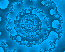David Milovich Mathematical images

Given a map f and initial point z in the complex plane, the sequence z,f(z),f(f(z)),f(f(f(z))),... is either bounded or not. So, fixing f and varying z, we obtain a partition of the complex plane into two sets. The boundary between them, called a Julia set, is usually fractal. (This is a bit of an oversimplification. See below.)

The links below are to Julia sets for various f(z). The initial points that appear to produce a bounded sequence are colored black. The other points are colored according to how long it takes the sequence to start looking like it's headed off to infinity.
Julia set of z^3 - i*z + 1/4
Julia set of z*sin(z) + 1/5
Julia set of z * (1 + (z - exp(1.4*i)) / 2); zooming in (different colors).

Actually, the definition of Julia set is a bit more subtle. Even if two initial points' associated sequences are both bounded, we still distinguish between them if the sequences converge to different points (or, more generally, to different limit sets). By making more distinctions, we may partition the complex plane into more regions. The Julia set is the union of the boundaries between these regions. Again, it is usually fractal

A Julia set for Newton's method is a Julia set for f(z) of the special form z-g(z)/g'(z). In nice regions, z,f(z),f(f(z)),... converges to a root of g. We color z according to which root, if any, the sequence converges to. For further reading, I recommend Simon Tatham's page.

Newton's method on z^6 - 1
Newton's method on z^7 - 1
Newton's method on z^7 + z / 1000 - 1
Newton's method on z^7 + i*z/100000 - 1
Newton's method on three slightly assymmetrical roots
Newton's method on seven slightly asymmetrical roots; zooming in; zooming in again (different colors).

The link below goes to a picture of a tiny fraction of the Mandelbrot set, which is a little different from a Julia set: z is fixed at 0 and f varies over the family of quadratic polynomials z^2+c. But there is again a fractal boundary between those c that produce a bounded sequence and those that don't.

Zooming in on the Mandelbrot set

I produced the above images back in the day using a Windows application that I wrote in VB6(!).